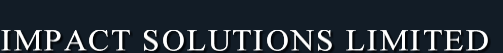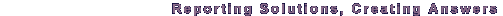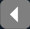Do your homework and know your facts, but remember it's passion that persuades# The Maximum Impact Solutions Blog09
June

## How Do I Create a Customed Grouped Financial Year Report in Crystal Reports?The delegate also wanted to be able to create a report that could be group by fiscal year, fiscal quarters and fiscal month, where their financial year started on the 1st of May.

To create this report, three formulas would have to be created:

•        Fiscal Year
•         Fiscal Qtrs, and
•         Fiscal Month

The financial fiscal year formula (@Fiscal Year):

If  Month({Tablename.DateTimeFieldname}) >= 5 then

Year ({Tablename.DateTimeFieldname})+1 else

Year ({Tablename.DateTimeFieldname})

The financial fiscal quarters formula (@Fiscal Qtrs):

If  Month({Tablename.DateTimeFieldname}) in [5,6,7] then “Qtr 1” else

If  Month({Tablename.DateTimeFieldname}) in [8,8,9] then “Qtr 2” else

If  Month({Tablename.DateTimeFieldname}) in [10,11,12] then “Qtr 3” else “Qtr 4”

The financial fiscal months formula (@Fiscal Months):

If  Month({Tablename.DateTimeFieldname}) > = 5  then

“Month ”& Totext(Month({Tablename.DateTimeFieldname}) – 4,’00’) else

“Month ”& Totext(Month({Tablename.DateTimeFieldname}) + 8,’00')

Add a group using the {@Fiscal Year} formula field

Add a second group using the {@Fiscal Qtrs} formula field

Add a third group using the {Fiscal Months} formula field

1

14
April
thanks bro....

### Name *